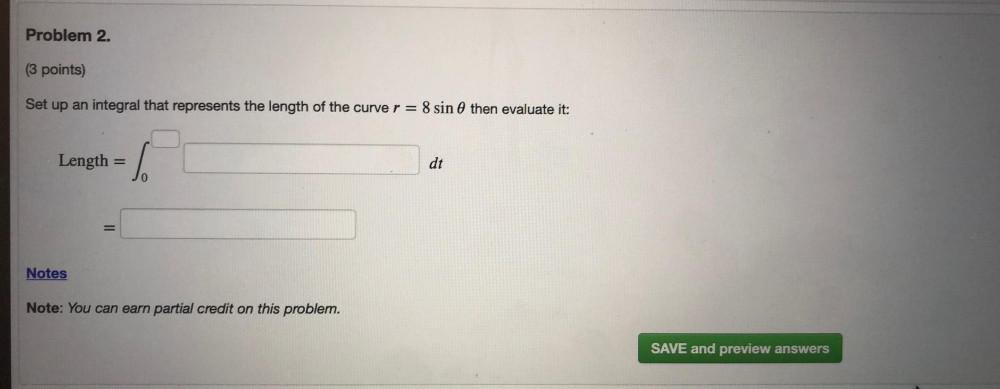Question:

# Problem 2. (3 points) Set up an integral that represents the length of the curve r = 8 sin @ then evaluate it: Length = dt = NotProblem 2. (3 points) Set up an integral that represents the length of the curve r = 8 sin @ then evaluate it: Length = dt = Notes Note: You can earn partial credit on this problem. SAVE and preview answers